Courses

# RD Sharma Solutions -Page No.14.4, Circles, Class 6, Maths Class 6 Notes | EduRev

## RD Sharma Solutions for Class 6 Mathematics

Created by: Abhishek Kapoor

## Class 6 : RD Sharma Solutions -Page No.14.4, Circles, Class 6, Maths Class 6 Notes | EduRev

The document RD Sharma Solutions -Page No.14.4, Circles, Class 6, Maths Class 6 Notes | EduRev is a part of the Class 6 Course RD Sharma Solutions for Class 6 Mathematics.
All you need of Class 6 at this link: Class 6

#### PAGE NO 14.4:

Question 1: Explain the following:
(i) Circle
(iii) Centre
(iv) Diameter
(v) Chord
(vi) Interior of a circle
ANSWER: (i) A circle is a set of all those points in a plane, whose distance from a fixed point remains constant.
(ii) Radius of a circle is a line segment with one end at its centre and the other end on the circle. (It is the constant distance between all the points on the circle and its centre.)
(iii) The centre of a circle is that fixed point from which all points remain at a constant distance.
(iv) Diameter of a circle is a line segment passing through the centre of a circle, and having its end points on the circle.
(v) A chord of a circle is a line segment with its end points lying on the circle.
(vi) Interior of a circle is a set of all those points which lie inside the circle.

Question 2:  Take a point on your notebook and draw circle of radii 4 cm, 3 cm and 6.5 cm, each having the sme centre O.
The given figure shows circles of radii 4 cm, 3 cm and 6.5 cm, respectively.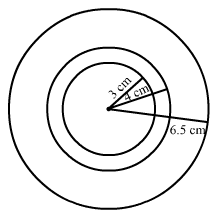Question 3: Draw a circle with centre O and any radius. Draw AC and BD two perpendicular diameters of the circle. Join AB, BC, CD and DA.
ANSWER: The figure is shown below -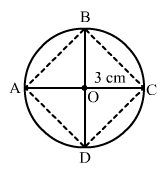#### Rewrite each of the following statements using the correct symbol (=,< or >):(i) OQ....5 cm(ii) OP....5 cm(iii) OR ....5 cmANSWER:The given figure shows the points P, Q and R such that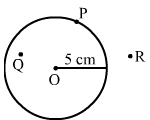(i) P lies on the circle.
(ii) Q lies in the interior of the circle.
(iii) R lies on the exterior of the circle.

(i) OQ < 5 cm
(ii) OP = 5 cm
(iii) OR> 5 cm

#### Question 5: Take two points A and B on the page of your note book. Draw a circle with centre A which passes through B.ANSWER: The figure is shown below -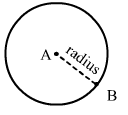Question 6: Draw a semi-circle with centre and radius 5 cm. Is the diameter that determines the semi-circle, a part of the semi-circle?

#### ANSWER:The semi -circle with centre O and radius 5 cm is shown below -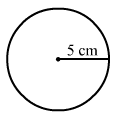The end point of a diameter of a circle divides it into two equal parts, and each part is called a semi circle. So, it is not the diameter, but end points of the diameter that determines the semi circle or a part of the semi circle.

103 docs

,

,

,

,

,

,

,

,

,

,

,

,

,

,

,

,

,

,

,

,

,

,

,

,

,

,

,

,

,

,

;